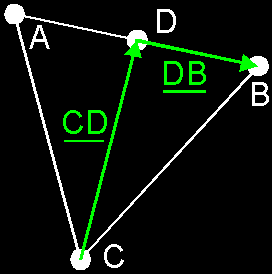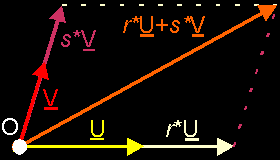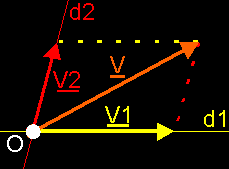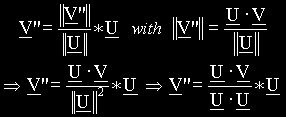3D Geometry Primer: Chapter 1 - Issue 03 - More On Vector Arithmetic by (28 August 2000) Return to The Archives
 More On Vector ArithmeticIn this issue, we will continue on with vector arithmetic. We'll use the stuff we learned last week to do more advanced operations with vectors. These are: linear combinations, decomposition of a vector, perpendicular projections and the triple product. We'll also discuss operator precedence.But first... the solution to last week's exercise. The exercise was to express the vectors CB, AD, DA, AB and CA as a combination of vectors CD and DB, using free vectors...And the answers are: CB = CD + DB (Just an addition of 2 vectors) AD = DB (Same direction, same sense, same length, thus same free vector) DA = –DB (Same direction, same length, but different sense). AB = 2*DB (Same direction, same sense, but twice as long) CA = CD – DB (see it as an additon of CD and –DB)

 (I) Advanced operationsThis is a combination of the addtion and scalar product. This is very important because it's used very often. To take a linear combination of 2 vectors, first scale both vectors U and V with the respective scalars r and s. You get r*U and s*V. Then add those results together and you will get: (r*U)+(s*V).Notice that the vectors r*U and s*V start at the origin too! In fact, all vectors start at the origin now.You can also do linear combinations with more vectors: r*U + s*V + t*W. This would be equal to (r*U)+(s*V)+(t*W).b) Decomposition Of A Vector:Say, you’ve got a vector V and two directions (lines) d1 and d2. You want to decompose this vector into two new vectors V1 and V2 with the given directions. (Why the ... would you want to do that?, you ask) That is, you want to find 2 vectors V1 and V2 that have the directions d1 and d2 respective, in such a way that if you add V1 and V2 together, you get V1+V2=V. Just create a parallelogram (the sides are parallel with the d1 and d2), and voilŕ, you have your 2 new vectors.c) Perpendicular Projection:If we have 2 vectors U and V, and we want to project V perpendicular on u (=the direction of U). How do we do that?Let's call the result vector V". If we look at the picture, we see that ||V"|| = ||V||*cos(theta), with theta the angle between U and V (trigonometry). This sounds familiar! It's a little bit like a dot product U·V = ||U||*||V||*cos(theta). If we look closer, we can see that ||V"|| = (U·V)/||U||. Now, how do we construct V"? We can see that V" has the same direction as U, so it should be possible to construct V" by a scalar product of U. Which scale should we take? We need to go from magnitude ||U|| to ||V"||, so we need to scale it with ||V"||/||U||. This results in: V" = (U·V)/||U||˛*U = (U·V)/(U·U)*UFor those people who weren't listening the last time: notice that the vector V" starts in the origin too. In fact, all vectors start in the origin!d) Mixed Product Or Triple Product: (U V W)This is an extra operator that I want to show you. It’s not so important, because it’s only a combination of the dot product and the cross product.definition: (U V W) = (U×V)·W = ||U||*||V||*||W||*sin(theta)*cos(phi)Interpretation: First, work out U×V and call the result Z . Z is a vector perpendicular to U and V. The magnitude of Z is equal to the area of the parallelogram between U and V: ||Z|| = ||U||*||V||*sin(theta). The original product is now simplified to Z·W . This dot product gives us a real value equal to ||Z||*||W||*cos(phi). We already know what ||Z|| is, and on the figure you can see that ||W||*cos(phi) is the height of the parallelepiped between U, V and W (kind of deformed beam). We know (at least I do :) that the volume of a parallelepiped is given by the base area multiplied with the height. Thus ||Z||*||W||*cos(phi) is the volume of that thing. Anyway: The mixed product (U V W) gives us the volume of the parallelepiped between U, V and W: ||U||*||V||*||W||*sin(theta)*cos(phi)Notice that: ° (U V W) = (V W U) = (W U V) = –(U W V) = –(V U W) = –(W V U) ° (r*U V W) = (U r*V W) = (U V r*W) = r*(U V W) ° (U V W) = 0 if the 3 vectors lay in 1 plane (the parallelepiped is flat).

 (II) Operator PrecedenceWhat about operator precedence? Well, like always, the operators + and – have lowest precedence.The other operations *, · and × are a bit tricky: the operators · and * have the same precedence: (r*U·V)=(r*U)·V=r*(U· V) . And so do × and * : r*U×V=(r*U)×V=r*(U×V) .Now I hear some of you think: "And thus · and × have the same precedence." Wrong! × has higher precedence than · ! (not very true, but it works...) I know this sounds very weird but it’s actually logical if you think a step further.Say we have the expression U·V×W and · and × had the same precedence. In that case, the following statement would be true: U·V×W = (U·V)×W = U·(V×W). This is total nonsense!!! First, (U·V) is a real value and you can not do a cross product on a real value and a vector. Second, one could say: "OK, if I have a real value and a vector, I simply use the scalar product: (U·V)*W." This isn’t justified either, because then you would get a vector at the end, and the other interpretation U·(V×W) would result in a real value. And there’s no way that a vector and a real value could be equivalent.Besides, you may not simply replace a cross product by a scalar product. Normally this isn't even possible, and I can’t think of a situation where it is allowed.Anyway: the only possible interpretation of U·V×W is U·(V×W) and we mostly will write this with the brackets, so that you won’t be confused. B.T.W.: have you noticed that U·V×W = (U V W)? It's a bit tricky, you have to shuffle with the vectors.Next week: we'll introduce vector bases and come a step closer to numerical vector arithmetic and "points". In the meantime: Sweat dreams are...Regards, Bramz